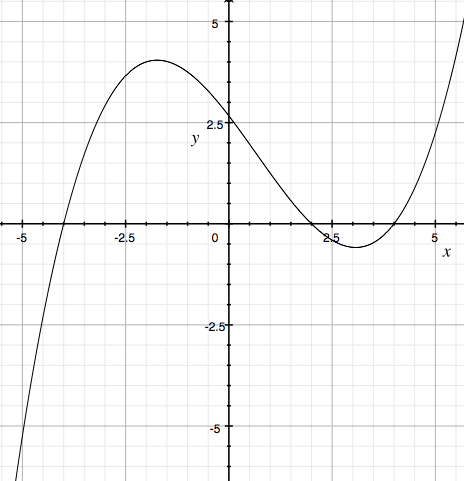SEARCH HOMEMath Central Quandaries & QueriesQuestion from Simran: what is difference between f(x) compared to f(-x) on graphHi Simran,

I want to illustrate using the graph of a function.Suppose $x = 2.5$ then from the graph it looks like $f(x) = f(2.5)$ is approximately $\large \frac12$ and $f(-x) = f(-2.5)$ is approximately $3 \large \frac{1}{2}.$ Also if $x=-5$ then $f(x) = f(-5)$ is a little less than $-5$ and $f(-x) = f(5)$ is a little less than $2 \large \frac{1}{2}.$

The point is that the relationship between $f(x)$ and $f(-x)$ depends very much on the value of $x$ and the shape of the graph.

I hope this helps,
Penny* Registered trade mark of Imperial Oil Limited. Used under license.Math Central is supported by the University of Regina and the Imperial Oil Foundation.